# 4. [10 points] Today is Nov 15. The following jobs need to be scheduled on the...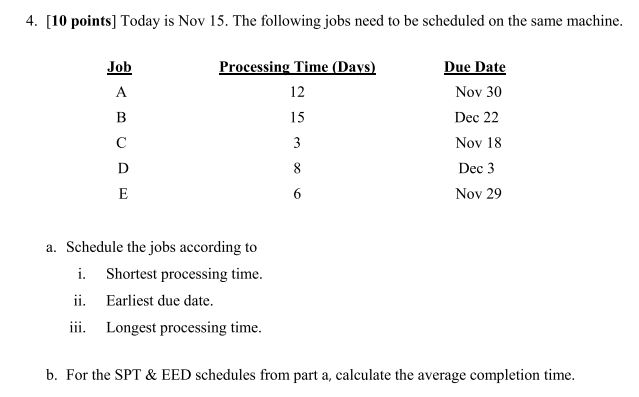4. [10 points] Today is Nov 15. The following jobs need to be scheduled on the same machine. Job Processing Time (Davs) Due Date Nov 30 Dec 22 Nov 18 Dec 3 Nov 29 12 a. Schedule the jobs according to i. Shortest processing time ii. Earliest due date. iii. Longest processing time. b. For the SPT& EED schedules from part a, calculate the average completion time.

The data provided in the question:-

 Job Processing time Due Date A 12 Nov 30 B 15 Dec 22 C 3 Nov 18 D 8 Dec 3 E 6 Nov 29

i:- Shortest processing time:- In order to find out the sequence as per this rule, the job which has the least processing time will be sequenced first and then the remaining jobs will be sequenced in the increasing processing time.

So the job sequence will be as below:-

C-E-D-A-B

ii:- Earliest due date:- In this, the job which has the least due date will be sequenced first followed by the jobs having later due dates.

So the sequence will be:-

C-E-A-D-B

iii:- Longest processing time:- In order to find out the sequence as per this rule, the job which has the maximum processing time will be sequenced first and then the remaining jobs will be sequenced in the decreasing processing time.

So the job sequence will be as below:-

B-A-D-E-C

Answer b:- For Shortest processing time:-

 Jobs Flow in Time Flow Out time C 0 0+3=3 E 3 3+6=9 D 9 9+8=17 A 17 17+12=29 B 29 29+15=44

Total Flow time = 3+9+17+29+44=102

Average completion time = Total flow time / number of jobs

Average completion time =102/5

Average completion time =20.4 Days

For Earliest Due Date:-

 Jobs Flow in Time Flow Out time C 0 0+3=3 E 3 3+6=9 A 9 9+12=21 D 21 21+8=29 B 29 29+15=44

Total Flow time = 3+9+21+29+44=106

Average completion time = Total flow time / number of jobs

Average completion time =106/5

Average completion time =21.2 Days

#### Earn Coin

Coins can be redeemed for fabulous gifts.

Similar Homework Help Questions
• ### ABC Enterprises has five jobs that must be scheduled for production at a work center. Below...

ABC Enterprises has five jobs that must be scheduled for production at a work center. Below are the processing times and due dates for each job.                                     Job Processing Time (in days) Due Date (days from today) 1 7 14 2 5 21 3 3 10 4 2 7 5 6 9                                                                                                                            Schedule the jobs by sequencing them using the Earliest Due Date (EDD) priority rule. What is the average flow time? Show your Work and round your...

• ### The following table contains information regarding jobs that are to be scheduled through one machine. Assume...

The following table contains information regarding jobs that are to be scheduled through one machine. Assume jobs are listed in order of arrival (i.e., A, then B, then C, etc.). JOB PROCESSING TIME (DAYS) DUE DATE     A 7 20     B 15 21     C 1 16     D 12 17     E 11 15     F 4 6     G 5 12 Round your answers to 1 decimal place. a. What is the first-come, first-served (FCFS) schedule? b. What is the shortest operating time...

• ### Problem 2 (6 points): Refer to the following data for jobs waiting to be processed at...

Problem 2 (6 points): Refer to the following data for jobs waiting to be processed at a single work center (jobs are shown in order of arrival): Job Processing Time (Days) Due Date (days from Now) A 2 2 B 5 6 C 6 10 D 9 6 E 4 8 What is the average flow time for the shortest processing time (SPT) rule schedule?

• ### a) Using the EDD (earliest due date) decision rule for sequencing the jobs, the order is...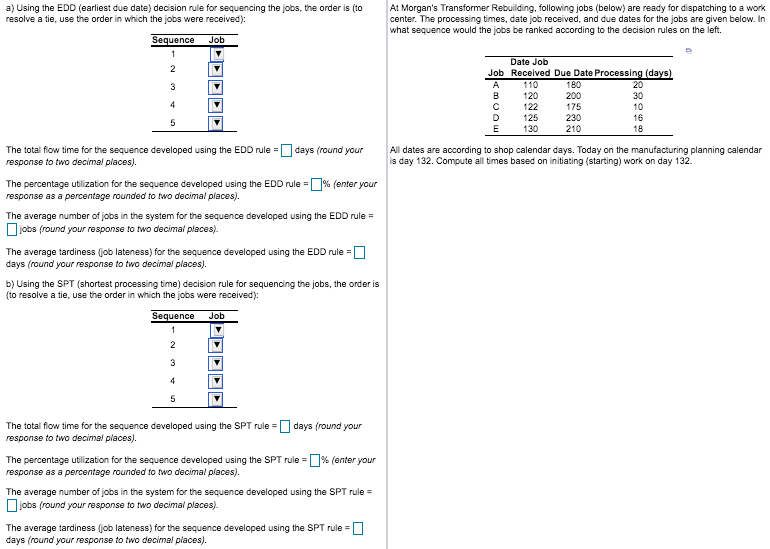a) Using the EDD (earliest due date) decision rule for sequencing the jobs, the order is (to resolve a tie, use the order in which the jobs were received): At Morgan's Transformer Rebuilding, following jobs (below) are ready for dispatching to a work center. The processing times, date job received, and due dates for the jobs are given below. In what sequence would the jobs be ranked according to the decision rules on the left Sequence Job 1 Date Job...

• ### a) Using the EDD (earliest due date) decision rule for sequencing the jobs, the order is...a) Using the EDD (earliest due date) decision rule for sequencing the jobs, the order is (to resolve a tie, use the order in which the jobs were received): At Morgan's Transformer Rebuilding, following jobs (below) are ready for dispatching to a work center. The processing times, date job received, and due dates for the jobs are given below. In what sequence would the jobs be ranked according to the decision rules on the left Sequence Job 1 Date Job...

• ### # A 3. The jobs listed below needed to be completed. Assume that it is now...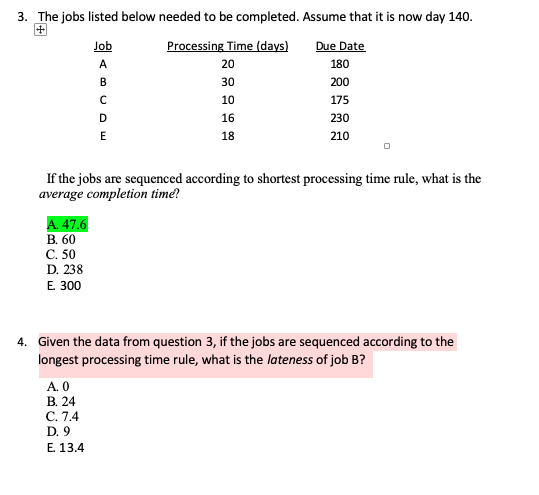# A 3. The jobs listed below needed to be completed. Assume that it is now day 140. Job Processing Time (days) Due Date 20 180 30 с 10 175 16 230 E 18 210 B 200 D 1:54 If the jobs are sequenced according to shortest processing time rule, what is the average completion time! A. 47.6 B. 60 C. 50 D. 238 E 300 4. Given the data from question 3, if the jobs are sequenced according to...

• ### What would be the sequence of the following jobs if you did them in Earliest Due...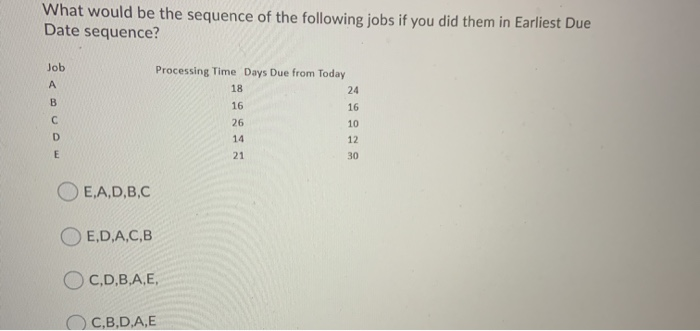What would be the sequence of the following jobs if you did them in Earliest Due Date sequence? Job A B Processing Time Days Due from Today 18 24 16 16 26 10 14 12 21 30 С D E OE,A,D,B,C E,D,A,C,B OC,D,B,A,E, C.B.D.A,E Question 6 (10 points) You have 5 jobs to do. What would be the sequence if you chose to do a Shortest Processing Time Approach? Job Processing Time Days Due from Today А 24 30 B...

• ### ​a) Using the SPT​ (shortest processing​ time) decision rule for sequencing the​ jobs, the order is​...

​a) Using the SPT​ (shortest processing​ time) decision rule for sequencing the​ jobs, the order is​ (to resolve a​ tie, use the order in which the jobs were​ received): Sequence Job 1 ▼ N L M K O 2 ▼ K O L M N 3 ▼ K N L O M 4 ▼ N L M O K 5 ▼ N O K M L The total flow time for the sequence developed using the SPT rule​ = nothing...

• ### Question 7 (10 points) What would be the sequence of the following jobs if you did...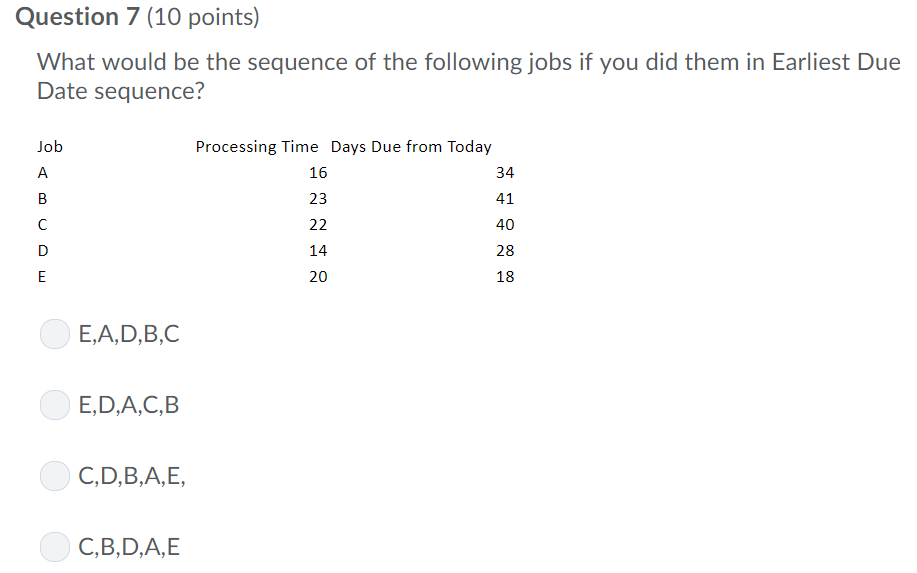Question 7 (10 points) What would be the sequence of the following jobs if you did them in Earliest Due Date sequence? Job Processing Time Days Due from Today 34 A 16 B 23 41 40 22 14 28 E 20 18 E,A,D,B,C E,D,A,C,B C,D,B,A,E C,B,D,A,E U Lu

• ### Five jobs are ready for processing at time zero through a workstation. The details of the...

Five jobs are ready for processing at time zero through a workstation. The details of the processing time and the due date of completion is provided in the following table. Job Processing Time (days) Due Date A 8 12 B 4 9 C 3 6 D 7 7 E 5 20 Using the shortest processing time (SPT) rule, it can be concluded that the average flow time for these five jobs is _____. a. more than 14 but less than...